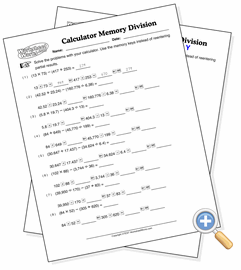# Subtraction with MemoryUse the memory feature to complete multi-step operations

These exercises are for helping students learn how to perform multi-step operations that involve finding the difference between the results of two other operations. For subtraction, the result of the first operation is added to memory, then the result of the second operation is subtracted from memory. The memory reveal button then shows what is the difference between the two. The other operations will not be subtraction, so the student needs to know how to perform all types of operations alone before learning the memory functions.